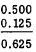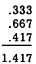Principles of MeasurementCustom SearchPRINCIPLES OF MEASUREMENT Computation with decimals frequently involves the addition or subtraction of numbers which do not have the same number of decimal places. For example, we may be asked to add such numbers as 4.1 and 32.31582. How should they be added? Should zeros be annexed to 4.1 until it is of the same order as the other decimal (to the same number of places)? Or, should .31582 be rounded off to tenths? Would the sum be accurate to tenths or hundred-thousandths? The answers to these questions depend on how the numbers originally arise. Some decimals are finite or are considered as such because of their use. For instance, the decimal that represents 1/2, that is 0.5, is as accurate at 0.5 as it is at 0.5000. Likewise, the decimal that represents 1/8 has the value 0.125 and could be written just as accurately with additional end zeros. Such numbers are said to be finite. Counting numbers are finite. Dollars and cents are examples of finite values. Thus, \$10.25 and \$5.00 are finite values. To add the decimals that represent 1/8 and 1/2, it is not necessary to round off 0.125 to tenths. Thus, 0.5 + 0.125 is added as follows:Notice that the end zeros were added to 0.5 to carry it out the same number of places as 0.125. It is not necessary to write such place-holding zeros if the figures are kept in the correct columns and decimal points are aligned. Decimals that have a definite fixed value may be added or subtracted although they are of different order. On the other hand, if the numbers result from measurement of some kind, then the question of how much to round off must be decided in terms of the precision and accuracy of the measurements. ESTIMATION Suppose that two numbers to be added resulted from measurement. Let us say that one number was measured with a ruler marked off in tenths of an inch and was found, to the nearest tenth of an inch, to be 2.3 inches. The other number measured with a precision rule was found, to the nearest thousandth of an inch, to be 1.426 inches. Each of these measurements requires estimation between marks on the rule, and estimation between marks on any measuring instrument is subject to human error. Experience has shown that the best the average person can do with consistency is to decide whether a measurement is more or less than halfway between marks. The correct way to state this fact mathematically is to say that a measurement made with an instrument marked off in tenths of an inch involves a maximum probable error of 0.05 inch (five hundredths is one-half of one tenth). By the same reasoning, the probable error in a measurement made with an instrument marked in thousandths of an inch is 0.0005 inch. PRECISION In general, the probable error in any measurement is one-half the size of the smallest division on the measuring �instrument. Thus the precision of a measurement depends upon how precisely the instrument is marked. It is important to realize that precision refers to the size of the smallest division on the scale; it has nothing to do with the correctness of the markings. In other words, to say that one instrument is more precise than another does not imply that the less precise instrument is poorly manufactured. In fact, it would be possible to make an instrument with very high apparent precision, and yet mark it carelessly so that measurements taken with it would be inaccurate. From the mathematical standpoint, the precision of a number resulting from measurement depends upon the number of decimal places; that is, a larger number of decimal places means a smaller probable error. In 2.3 inches the probable error is 0.05 inch, since 2.3 actually lies somewhere between 2.25 and 2.35. In 1.426 inches there is a much smaller probable error of 0.0005 inch. If we add 2.300 + 1.426 and get an answer in thousandths, the answer, 3.726 inches, would appear to be precise to thousandths; but this is not true since there was a probable error of .05 in one of the addends. Also 2.300 appears to be precise to thousandths but in this example it is precise only to tenths. It is evident that the precision of a sum is no greater than the precision of the least precise addend. It can also be shown that the precision of a difference is no greater than the less precise number compared.  To add or subtract numbers of different orders, all numbers should first be rounded off to the order of the least precise number. In the foregoing example, 1.426 should be rounded to tenths-that is, 1.4. This rule also applies to repeating decimals. Since it is possible to round off a repeating decimal at any desired point, the degree of precision desired should be determined and all repeating decimals to be added should be rounded to this level. Thus, to add the decimals generated by 1/3, 2/3, and 5/12 correct to thousandths, first round off each decimal to thousandths, and then add, as follows:When a common fraction is used in recording the results of measurement, the denominator of the fraction indicates the degree of precision. For example, a ruler marked in sixty-fourths of an inch has smaller divisions than one marked in sixteenths of an inch. Therefore a measurement of 3 4/64 inches is more precise than a measure of 3 1/16 inches, even though the 16 two fractions are numerically equal. Remember that a measurement of 3 4/64 inches contains a probable error of only one-half of one sixty-fourth of an inch. On the other hand, if the smallest division on the ruler is one-sixteenth of an inch, then a measurement of 3 1/16 inches contains a probable error of one thirty-second of an inch. ACCURACY Even though a number may be very precise, which indicates that it was measured with an instrument having closely spaced divisions, it may not be very accurate. The accuracy of a measurement depends upon the relative size of the probable error when compared with the quantity being measured. For example, a distance of 25 yards on a pistol range may be measured carefully enough to be correct to the nearest inch. Since there are 900 inches in 25 yards, this measurement is between 899.5 inches and 900.5 inches. When compared with the total of 900 inches, the 0.5-inch probable error is not very great. On the other hand, a length of pipe may be measured rather precisely and found to be 3.2 inches long. The probable error here is 0.05 inch, and this measurement is thus more precise than that of the pistol range mentioned before. To compare the accuracy of the two measurements, we note that 0.05 inch out of a total of 3.2 inches is the same as 0.5 inch out of 32 inches. Comparing this with the figure obtained in the other example (0.5 inch out of 900), we conclude that the more precise measurement is actually the less accurate of the two measurements considered. It is important to realize that the location of the decimal point has no bearing on the accuracy of the number. For example, 1.25 dollars represents exactly the same amount of money as 125 cents. These are equally accurate ways of representing the same quantity, despite the fact that the decimal point is placed differently. Practice problems. In each of the following problems, determine which number of each pair is more accurate and which is more precise: 1. 3.72 inches or 2,417 feet 2. 2.5 inches or 17.5 inches 3. 5 3/4: inches or 12 7/8: inches 4. 34.2 seconds or 13 seconds Answers: 1. 3.72 inches is more precise.     2,417 feet is more accurate. 2. The numbers are equally precise.     17.5 inches is more accurate. 3. l2 7/8; inches is more precise and more accurate. 4. 34.2 seconds is more precise and more accurate.Integrated Publishing, Inc. - A (SDVOSB) Service Disabled Veteran Owned Small Business